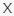# Math Trivia #1 TriviaBandit

Q 1. Let´s warm up…how much is 2 x 2 ÷ 2Choices:

1
4
5
2

2

Q 2. How much is 24 + 36 x 5Choices:

156
300
204
185

204

Q 3. In Roman Numerals, what does XL equate to?Choices:

90
15
60
40

40

Q 4. What is the area of a circle with a diameter of 20 inches if π= 3.1415?Choices:

380.1215 INCHES
3141.5 INCHES
314.15 INCHES
1256.6 INCHES

314.15 INCHES

Q 5. What is the square root of 49?Choices:

4
9
6
7

7

Q 6. How many sides does a trapezium have?Choices:

6
4
5
3

4

Q 7. Which Greek letter is used to signify summation?Choices:

DELTA
ALPHA
SIGMA
OMEGA

SIGMA

Q 8. Continue this pattern: 144, 121, 100, 81, 64Choices:

54
32
42
49

49

Q 9. Which of these is a perfect number?Choices:

36
6
102
9

6

Q 10. How many sides of equal length does an isosceles triangle have?Choices:

3
0
2
1